NCERT Solutions for Class 10 Maths Chapter 14 Statistics Ex 14.3 are part of NCERT Solutions for Class 10 Maths. Here we have given NCERT Solutions for Class 10 Maths Chapter 14 Statistics Ex 14.3.

 Board CBSE Textbook NCERT Class Class 10 Subject Maths Chapter Chapter 14 Chapter Name Statistics Exercise Ex 14.3 Number of Questions Solved 7 Category NCERT Solutions

## NCERT Solutions for Class 10 Maths Chapter 14 Statistics Ex 14.3

Question 1.
The following frequency distribution gives the monthly consumption of electricity of 68 consumers of a locality. Find the median, mean and mode of the data and compare them.Solution:Question 2.
If the median of the distribution given below is 28.5, find the values of x and y.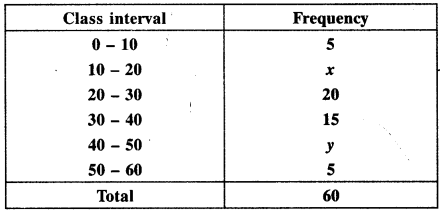Solution:
Given, Median = 28.5 which lies in the class (20 – 30).
Here, l = 20, f = 20, cf = 5 + x, h = 10, n = 60Question 3.
A life insurance agent found the following data for distribution of ages of 100 policy holders. Calculate the median age, if policies are given only to persons having age 18 years onwards but less than 60 years.Solution: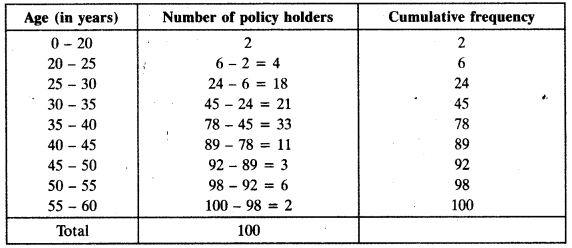Question 4.
The lengths of 40 leaves of a plant are measured correct to nearest millimetre, and the data obtained is represented in the following table: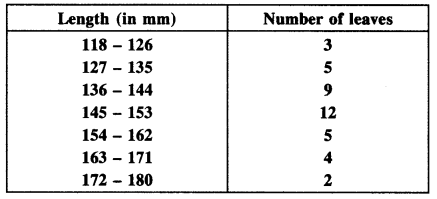Find the median length of the leaves.
(Hint: The data needs to be converted to continuous classes for finding the median since the formula assumes continuous classes. The classes then change to 117.5 – 126.5,126.5 -135.5,…, 171.5 -180.5.)
Solution: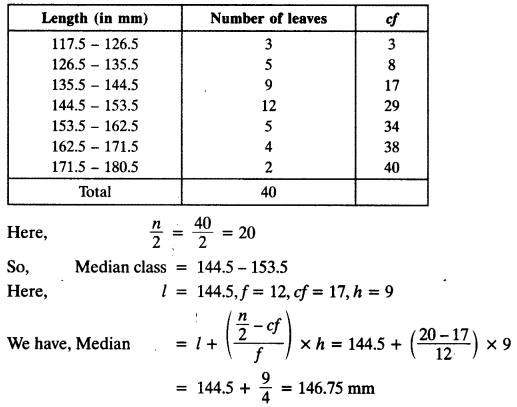Question 5.
The following table gives the distribution of the lifetime of 400 neon lamps: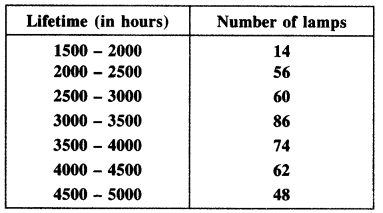Find the median lifetime of a lamp.
Solution: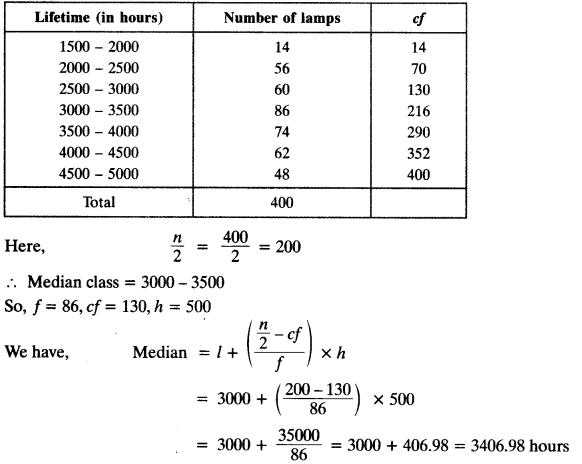Question 6.
100 surnames were randomly picked up from a local telephone directory and the frequency distribution of the number of letters in the English alphabet in the surnames was obtained as follows:Determine the median number of letters in the surnames. Find the mean number of letters in the surnames. Also, find the modal size of the surnames.
Solution: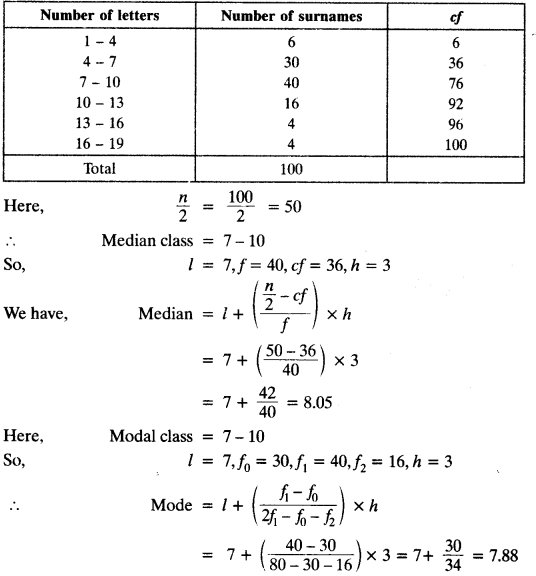For mean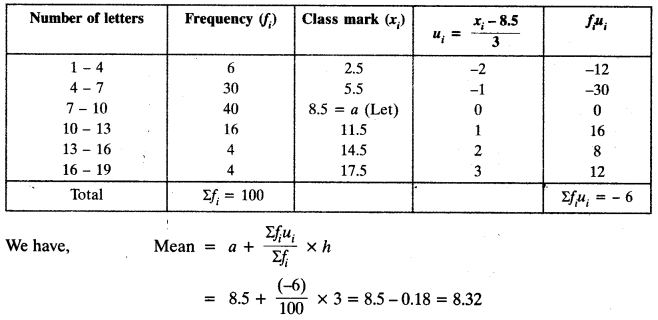Question 7.
The distribution below gives the weight of 30 students of a class. Find the median weight of the students.Solution: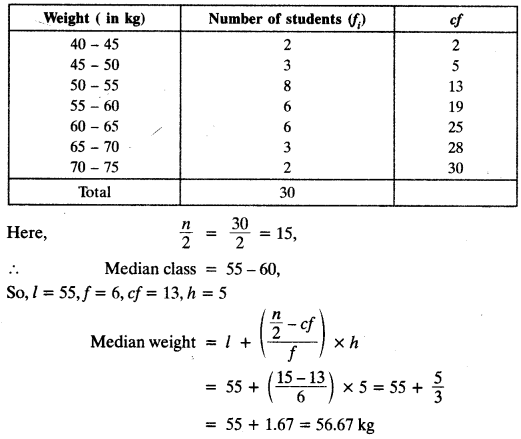We hope the NCERT Solutions for Class 10 Maths Chapter 14 Statistics Ex 14.3, help you. If you have any query regarding NCERT Solutions for Class 10 Maths Chapter 14 Statistics Ex 14.3, drop a comment below and we will get back to you at the earliest.